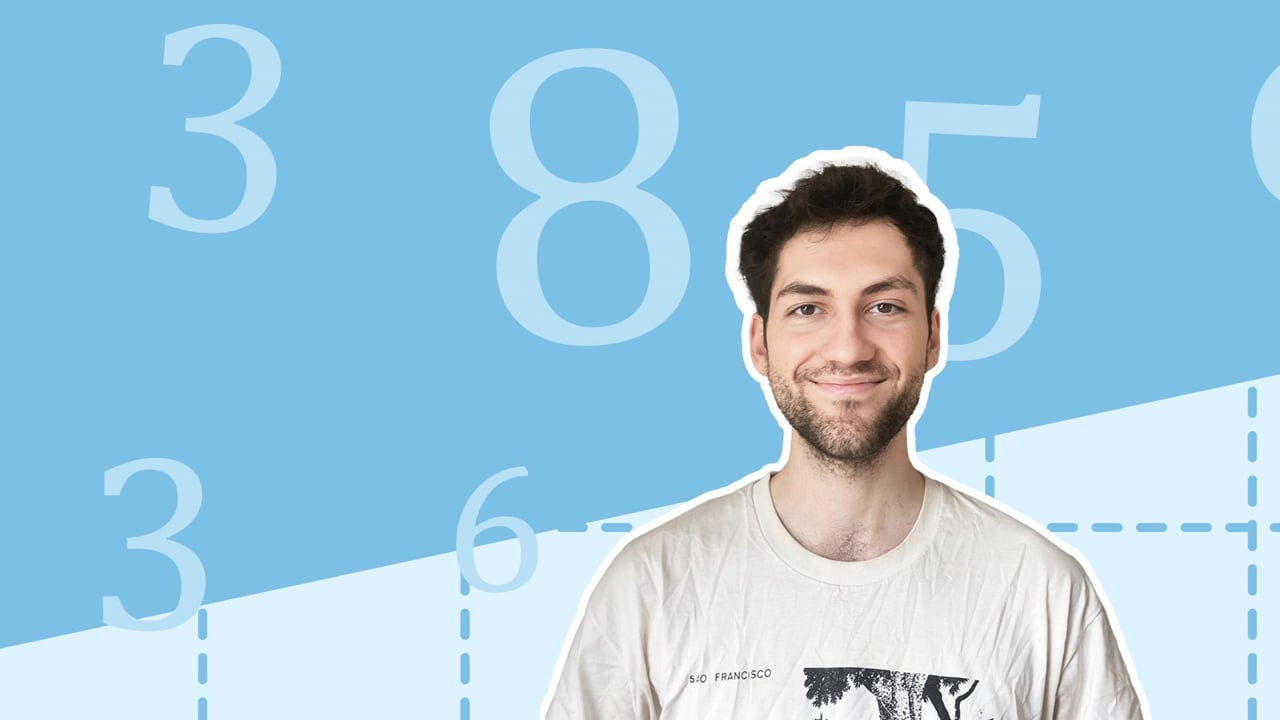Chapter OverviewMaths

Number and place value

Multiplication and division

Fractions

Measurement

Geometry - properties of shapes

Geometry - position and direction

Statistics

Ratio and proportion

Algebra

Maths

# Dividing fractions by whole numbers0%

Summary

# Dividing fractions by whole numbers

## ​​In a nutshell

Dividing fractions by whole numbers is a case of either multiplying the denominator of the fraction by the whole number, or dividing the numerator of the fraction by the whole number - whichever is easier! Always try to simplify at the end.

## Fractions reminder

Remember that a fraction represents one number divided by another number, specifically, the numerator divided by the denominator. So

$\dfrac49 = 4 \div 9$​​

There are two different methods you can use to divide a fraction by a whole number.

### Multiply the denominator

#### Procedure

 1 Leave the numerator as it is. 2 Multiply the denominator by the whole number you are dividing by. 3 Simplify the fraction if necessary.
##### Example 1

Solve the following question:

$\dfrac49\div2$​​

Leave the numerator as it is, and multiply the denominator by $2$​.

$\dfrac49\div2 = \dfrac{4}{9\times2}=\dfrac{4}{18}$​​

Simplify the fraction (if necessary) to reach the final answer.

$\dfrac{4}{18}=\underline{\dfrac{2}{9}}$​​​​

### Divide the numerator

#### Procedure

 1 Leave the denominator as it is. 2 Divide the numerator by the whole number you are dividing by. 3 Simplify the fraction if necessary.

##### Example 2

Solve the following question:

$\dfrac49\div2$​​

Leave the denominator as it is, and divide the numerator by $2$​.

$\dfrac49 \div 2 = \dfrac{4\div2}{9} = \underline{\dfrac{2}{9}}$​​

Note: As you can see, in this case, the second method was quicker as there was no simplifying needed.  However, both methods resulted in the same answer.

FAQs

• Question: How can you divide a fraction by a whole number?

Answer: You can also divide a fraction by a whole number by multiplying the denominator of the fraction by the whole number and simplifying.

• Question: How can you divide a fraction by a whole number?

Answer: Dividing fractions by whole numbers is a case of dividing the numerator of the fraction by the whole number and simplifying.

Theory

Exercises